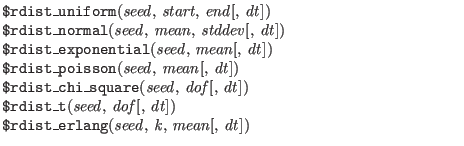Next: Other System Functions Recognized Up: System Tasks Previous: Simulation Control   Contents   Index

#### Random Numbers

$random[(seed)]$random generates a random integer every time it is called. If the sequence is to be repeatable, the first time one invokes $random it is given a numerical argument (a seed). Otherwise the seed is derived from the computer clock.In WRspice, the following functions are implemented in such a way that they are compatible with Newton iterations and convergence testing. Logically, a separate random value is obtained at each point of a grid in time that covers the simulation interval. The actual random number used is interpolated from this grid at the present simulation time. Thus, the random'' function becomes deterministic, and simulations that include output from the random generator will converge and iterate normally. This can be used to model Johnson noise in the time domain, for example. Each has an additional optional dt'' argument which if given is taken as the time period of the random number grid. If not given, the TStep from the running transient analysis is assumed. This value has significance only in transient analysis. During other types of analysis, calls to these functions will return a single random value, generated on the first call. Note that during transient analysis, the seed value should not change, or non-convergence can result. The following rules apply to these functions. 1. All arguments to the system functions are real values, except for seed (which is defined by$random). For the $rdist_exponential,$rdist_poisson, $rdist_chi_square,$rdist_t, and $rdist_erlang functions, the arguments mean, dof, and k shall be greater than zero (0). 2. Each of these functions returns a pseudo-random number whose characteristics are described by the function name, e.g.,$rdist_uniform returns random numbers uniformly distributed in the interval specified by its arguments.

3. For each system function, the seed argument shall be an integer. If it is an integer variable, then it is an inout argument; that is, a value is passed to the function and a different value is returned. The variable is initialized by the user and only updated by the system function. This ensures the desired distribution is achieved upon successive calls to the system function. If the seed argument is a parameter or constant, then the system function does not update the value. This makes the system function useable for parameter initialization. WRspice doesn't handle this.

4. The system functions shall always return the same value given the same seed. This facilitates debugging by making the operation of the system repeatable. In order to get different random values when the seed argument is a parameter, the user can override the parameter.

The two paragraphs above are difficult to follow. In WRspice, if the same seed value is used for all calls, the sequence of values is repeatable. A call with a different seed will reset the internal random number generator and a different sequence would be returned. The system functions never reset the seed. There is only one seed in WRspice, so if any function call changes the seed, all subsequent random number calls are affected.

5. All functions return a real value.

6. In $rdist_uniform, the start and end arguments are real inputs which bound the values returned. The start value shall be smaller than the end value. 7. The mean argument used by$rdist_normal, $rdist_exponential,$rdist_poisson, and $rdist_erlang is a real input which causes the average value returned by the function to approach the value specified. 8. The standard_deviation argument used by$rdist_normal is a real input, which helps determine the shape of the density function. Using larger numbers for standard_deviation spreads the returned values over a wider range. Using a mean of zero (0) and a standard deviation of one (1), $rdist_normal generates Gaussian distribution. 9. The dof (degree of freedom) argument used by$rdist_chi_square and \$rdist_t is a real input, which helps determine the shape of the density function. Using larger numbers for dof spreads the returned values over a wider range.Next: Other System Functions Recognized Up: System Tasks Previous: Simulation Control   Contents   Index
Stephen R. Whiteley 2022-09-18